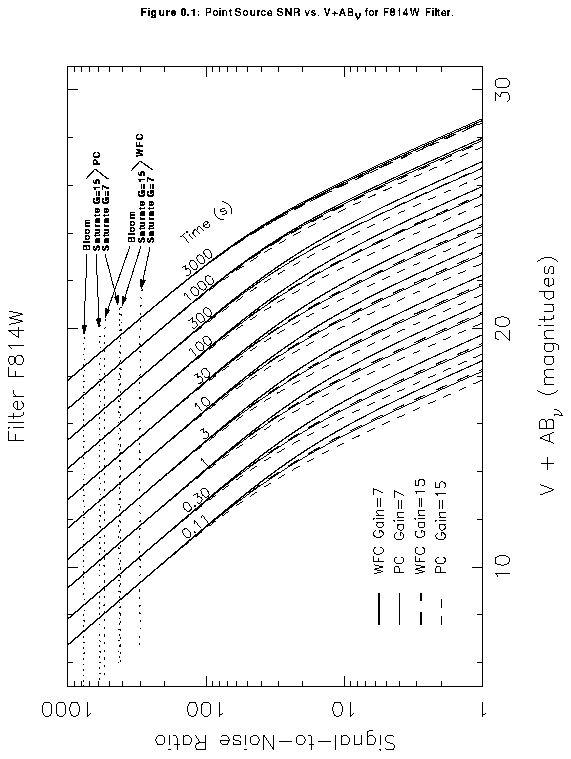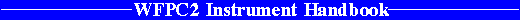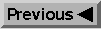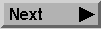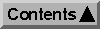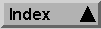Point Source SNR Plots

# B.1 Plots for Estimating Point Source SNR

In this section we present plots which may be used to rapidly estimate signal-to-noise ratio (SNR) for point sources with stellar spectra observed through popular WFPC2 filters. Plots are given for filters F160BW, F218W, F255W, F300W, F336W, F410M, F439W, F502N, F547M, F555W, F606W, F675W, F702W, and F814W. These plots assume that the data will be analyzed by PSF fitting, which optimizes the SNR in the background noise limited case. They also assume an average sky background of V=22.9 mag arcsec^-2. These plots do not explicitly include SNR reduction due to CR-SPLITing when the read noise dominates, but this effect is easily included as described below.

In situations requiring more detailed calculations (non-stellar spectra, extended sources, other sky background levels, don't know target V magnitude, etc.), the WFPC2 Exposure Time Calculator tool, located on the WFPC2 WWW pages, should be used instead.

One uses these plots as follows:

1. Examine Table B.1 and find the spectral type and wavelength of the desired filter (e.g. F555W xbb 5500Å). Interpolate in the Table to get ABn.

2. Sum the V magnitude of the target and AB_n derived from the Table.

3. Find the appropriate plot for the filter in question, and locate V+AB_n on the horizontal axis. Then read off the SNR for the desired exposure time, or vice-versa.

4. To get accurate values for CR-SPLIT exposures, one should use the sub-exposure time when consulting the plot, and then multiply the resulting SNR by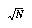, where N is the number of sub-exposures to be averaged.

There are separate lines for PC1 (light lines) and the WFC (heavy lines), as well as for ATD-GAIN=7 (default, solid lines), and ATD-GAIN=15 (dashed lines).

Dotted lines across the top of each plot indicate the onset of saturation. There are lines for saturation of the ATD-GAIN=7 setting (G=7), saturation of the ATD-GAIN=15 setting (G=15), and finally a line where blooming starts (top-most line for given camera).

We now give a sample SNR calculation using these plots. Consider a V=20 star of spectral class G0, for which we want to derive the SNR for 1200s CR-SPLIT exposure in F555W on PC1. We look up the G0 spectral class and F555W filter (5500Å) in Table B.1, and obtain ABn=0.02. We thus have V+ABn=20.02. We look at Figure B.10 and find this value on the horizontal axis. We locate exposure time 600s (one-half of the total 1200s CR-SPLIT exposure), and find SNR~200. For the total 1200s exposure the SNR would be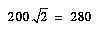. This exposure is well below the saturation lines in the plot, so saturation is not a concern. If instead, the star had V=19, we would be approaching the "Saturate G=7 PC" line indicating A-to-D converter saturation at gain 7, and so would want to specify ATD-GAIN=15.

#### Table B.1: AB_nu as a Function of Wavelength.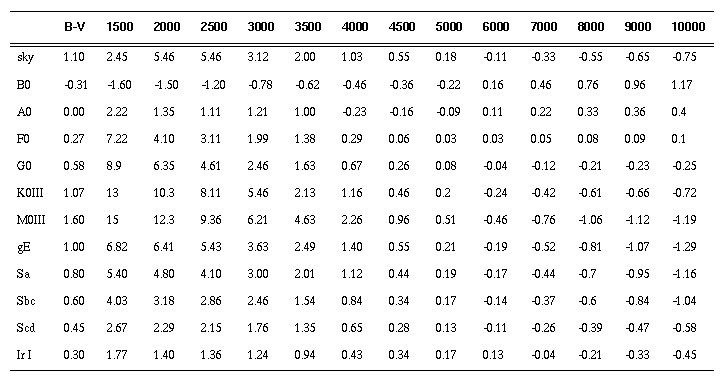#### Figure B.1: Point Source SNR vs. V+AB_nu for F160BW Filter.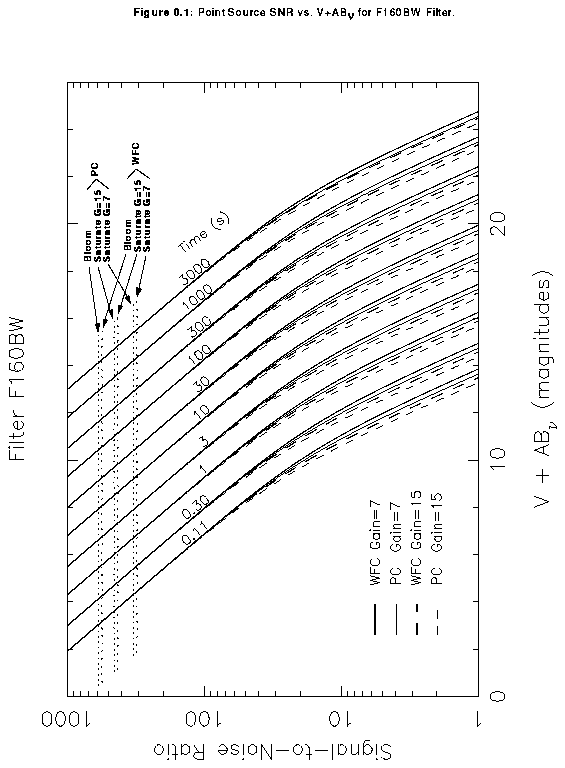#### Figure B.2: Point Source SNR vs. V+AB_nu for F218W Filter.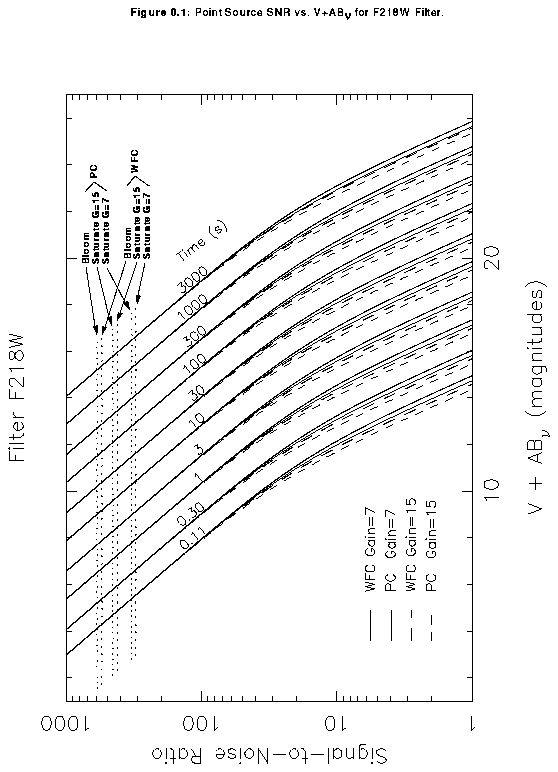#### Figure B.3: Point Source SNR vs. V+AB_nu for F255W Filter.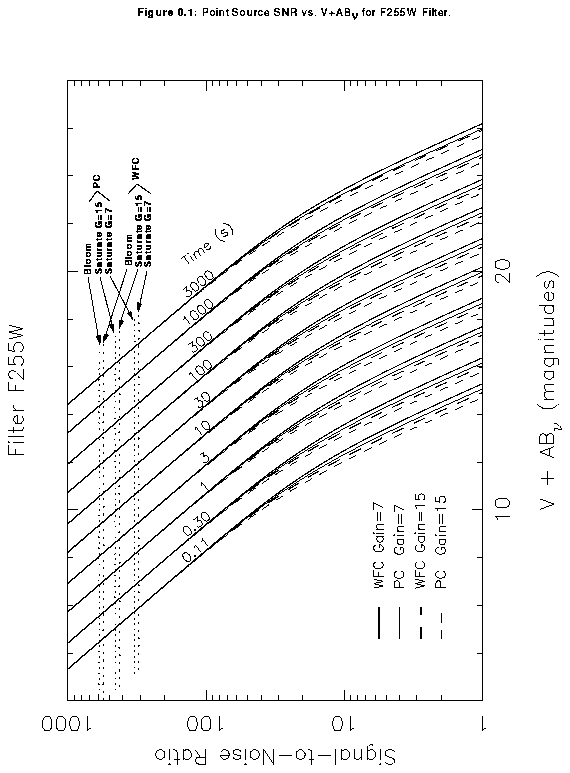#### Figure B.4: Point Source SNR vs. V+AB_nu for F300W Filter.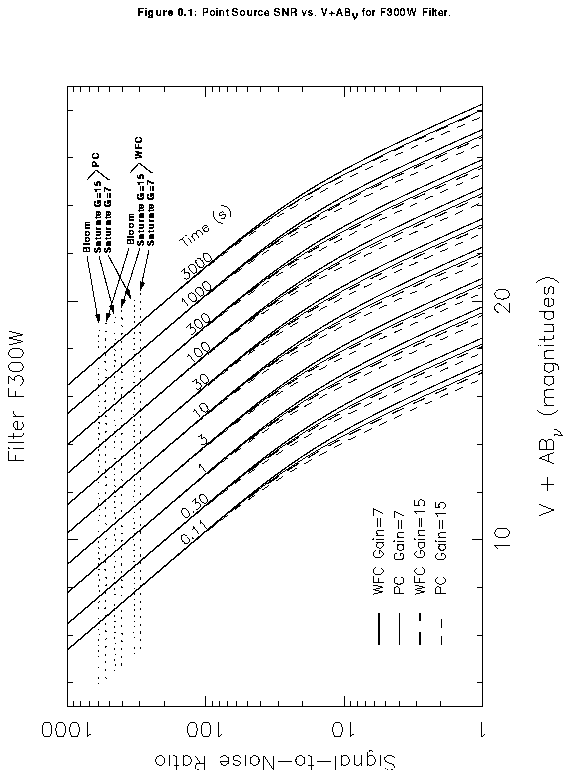#### Figure B.5: Point Source SNR vs. V+AB_nu for F336W Filter.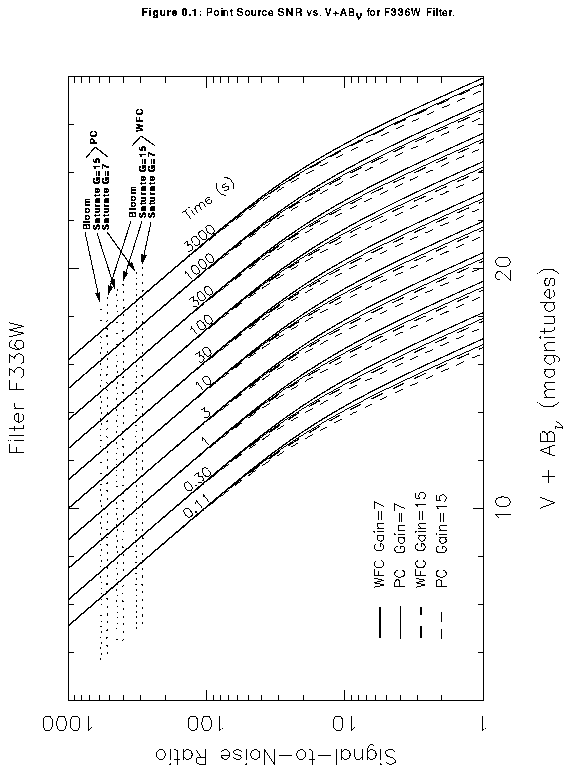#### Figure B.6: Point Source SNR vs. V+AB_nu for F410M Filter.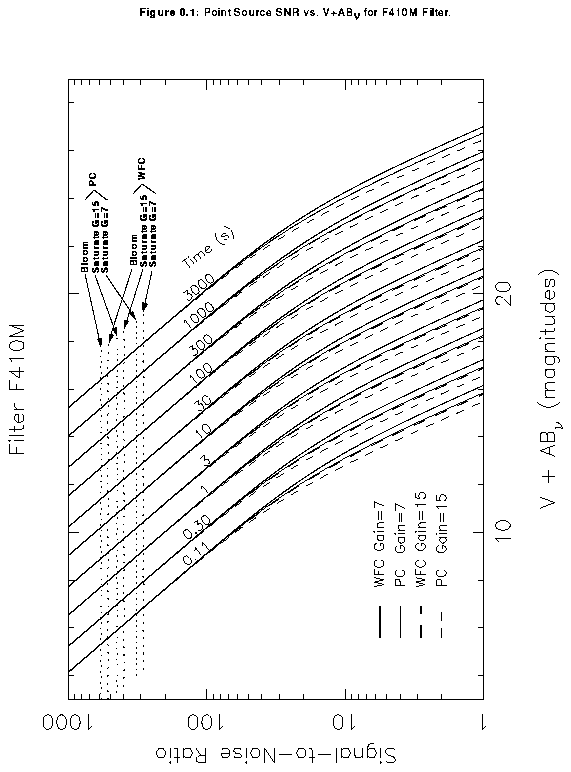#### Figure B.7: Point Source SNR vs. V+AB_nu for F439W Filter.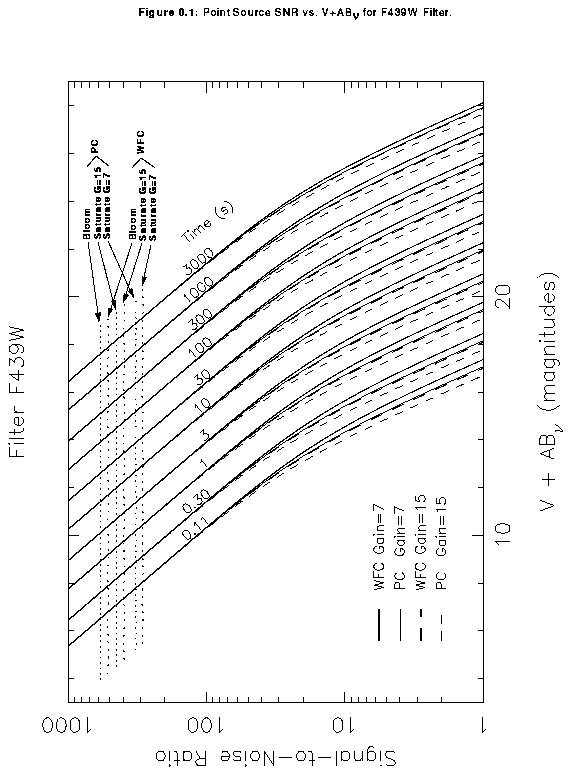#### Figure B.8: Point Source SNR vs. V+AB_nu for F502N Filter.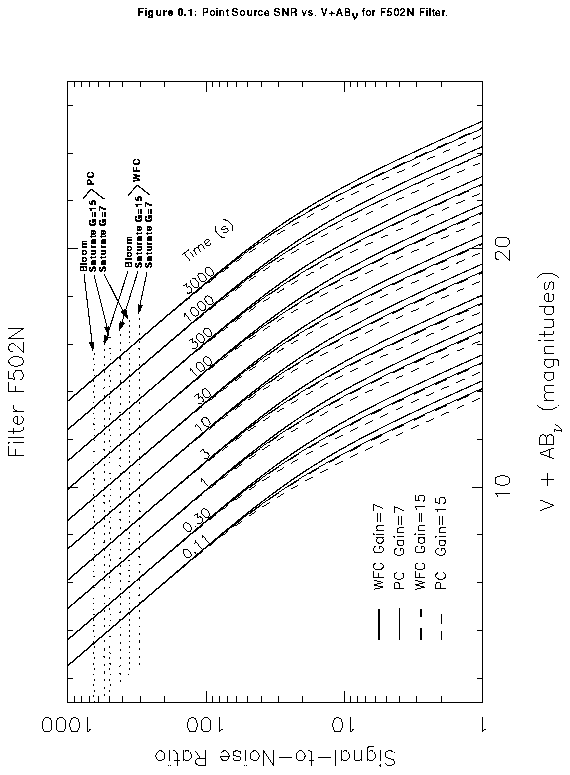#### Figure B.9: Point Source SNR vs. V+AB_nu for F547M Filter.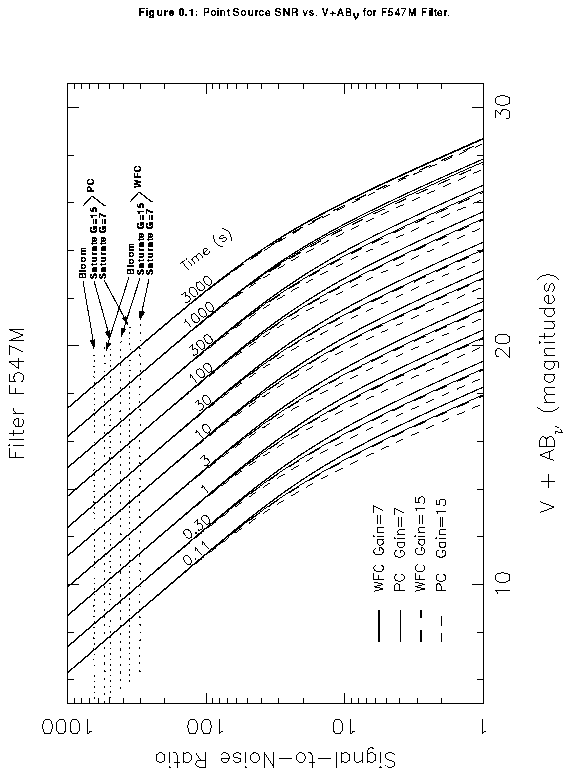#### Figure B.10: Point Source SNR vs. V+AB_nu for F555W Filter.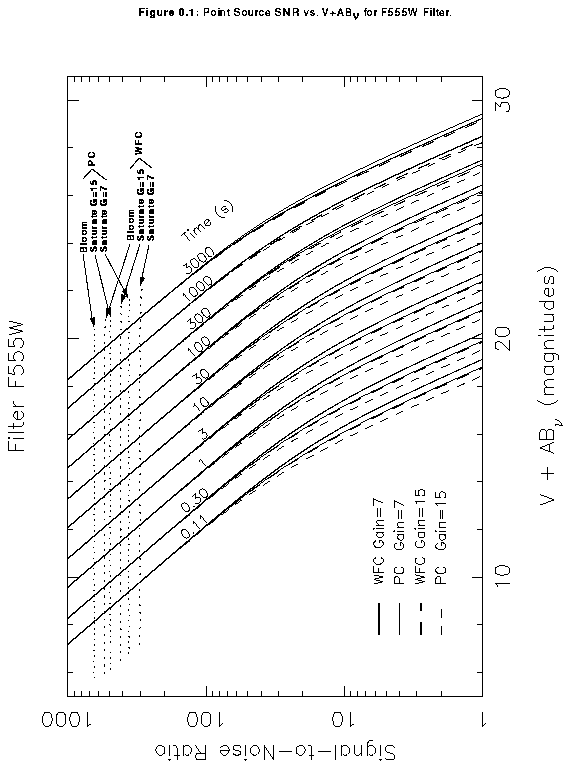#### Figure B.11: Point Source SNR vs. V+AB_nu for F606W Filter.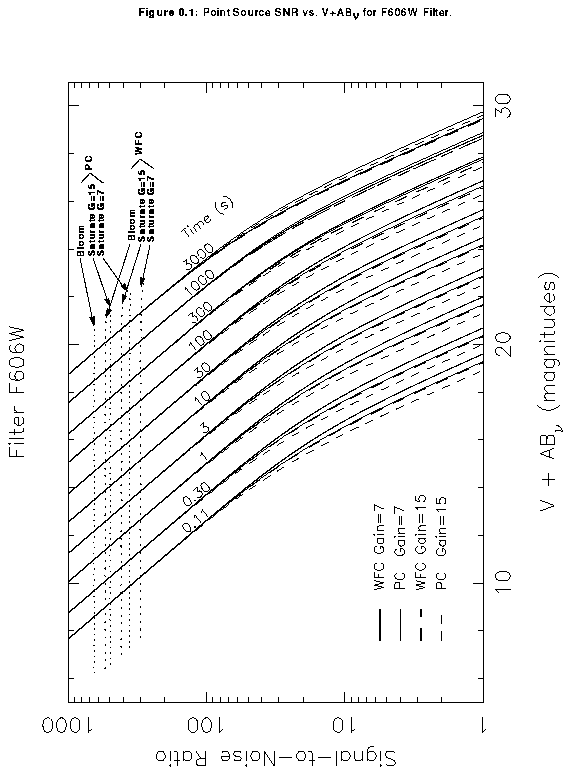#### Figure B.12: Point Source SNR vs. V+AB_nu for F675W Filter.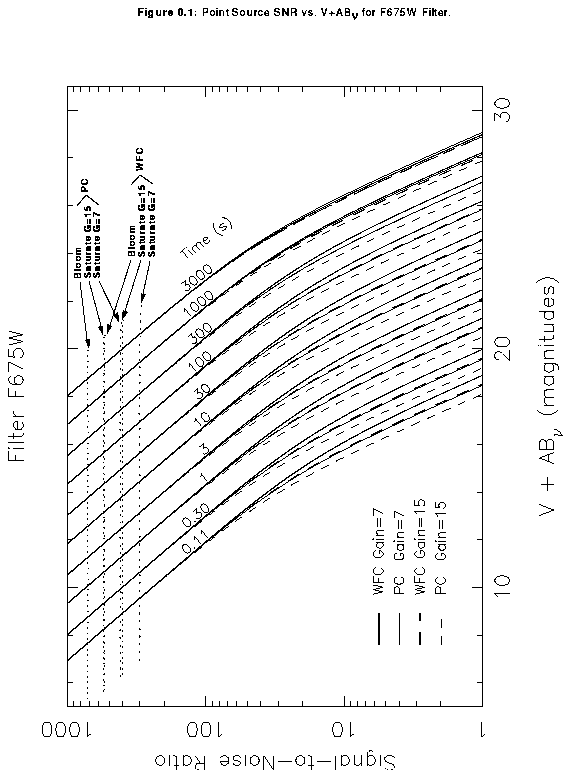#### Figure B.13: Point Source SNR vs. V+AB_nu for F702W Filter.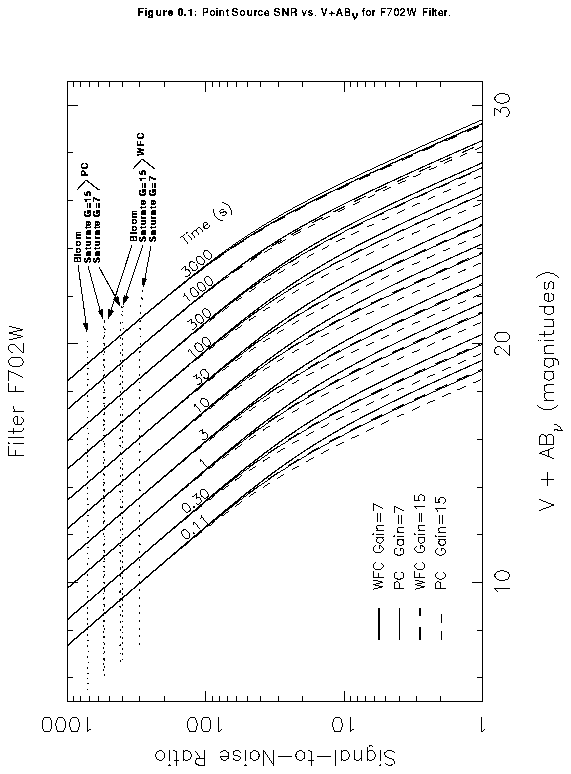#### Figure B.14: Point Source SNR vs. V+AB_nu for F814W Filter.